# Match the following sets for all sets A, B and C. Column A Column B (i) ((A′ ∪ B′) – A)′ (a) A – B (ii) [B′ ∪ (B′ – A)]′ (b) A (iii) (A – B) – (B – C) (c) B (iv) (A – B) ∩ (C – B) (d) (A × B) - Mathematics

Match the Columns

Match the following sets for all sets A, B, and C.

 Column A Column B (i) ((A′ ∪ B′) – A)′ (a) A – B (ii) [B′ ∪ (B′ – A)]′ (b) A (iii) (A – B) – (B – C) (c) B (iv) (A – B) ∩ (C – B) (d) (A × B) ∩ (A × C) (v) A × (B ∩ C) (e) (A × B) ∪ (A × C) (vi) A × (B ∪ C) (f) (A ∩ C) – B

#### Solution

 Column A Answer (i) ((A′ ∪ B′) – A)′ (b) A (ii) [B′ ∪ (B′ – A)]′ (c) B (iii) (A – B) – (B – C) (a) A – B (iv) (A – B) ∩ (C – B) (f) (A ∩ C) – B (v) A × (B ∩ C) (d) (A × B) ∩ (A × C) (vi) A × (B ∪ C) (e) (A × B) ∪ (A × C)

Explanation:

(i) ((A′ ∪ B′) – A)′

[(A′ ∪ B′) – A]′

= [(A′ ∪ B′) ∩ A’]’  ......{∵ A – B = A ∩ B’}

= [(A ∩ B)’ ∩ A’]’  ......{∵ (A ∩ B)’ = A’ ∪ B’}

= [(A ∩ B)’]’ ∪ (A’)’

= (A ∩ B) ∪ A

= A

((A′ ∪ B′) – A)′ = A

(ii) [B′ ∪ (B′ – A)]′

[B′ ∪ (B′ – A)]′

= (B’)’ ∩ (B’ – A)’  ......{∵ (A ∪ B)’ = A’ ∩ B’}

= B ∩ (B’ ∩ A’)’ ......{∵ A – B = A ∩ B’}

= B ∩ [(B’)’ ∪ (A’)’] ......{∵ (A ∩ B)’ = A’ ∪ B’}

= B ∩ (B ∪ A)

= B

[B′ ∪ (B′ – A)]′ = B

(iii) (A – B) – (B – C)

Step 1: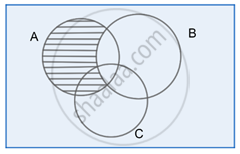A – B

Step 2: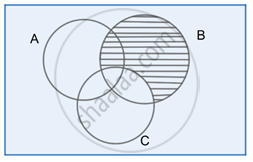B – C

Step 3: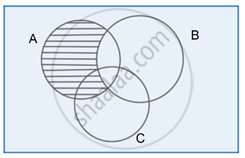(A – B) – (B – C)

Clearly, the Venn diagram in Step 3 shows same region as in Step 1

Hence, (A – B) – (B – C) = A – B

(iv) (A – B) ∩ (C – B)

Step 1: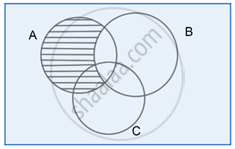A – B

Step 2: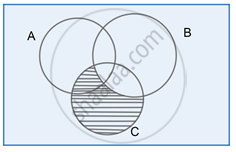C – B

Step 3: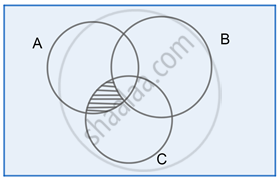(A – B) ∩ (B – C)

Clearly, from the Venn diagram in Step 3:

(A – B) ∩ (B – C) = A ∩ C – B

(v) A × (B ∩ C)

Let y ∈ A × (B ∩ C)

⇒ y ∈ A and y ∈ (B ∩ C)

⇒ y ∈ A and (y ∈ B and y ∈ C)

⇒ (y ∈ A and y ∈ B) and (y ∈ A and y ∈ C)

⇒ y ∈ (A × B) and y ∈ (A × C)

⇒ y ∈ (A × B) ∩ (A × C)

A × (B ∩ C) = (A × B) ∩ (A × C)

(vi) A × (B ∪ C)

Let y ∈ A × (B ∪ C)

⇒ y ∈ A and y ∈ (B ∪ C)

⇒ y ∈ A and (y ∈ B or y ∈ C)

⇒ (y ∈ A and y ∈ B) or (y ∈ A and y ∈ C)

⇒ y ∈ (A × B) or y ∈ (A × C)

⇒ y ∈ (A × B) ∪ (A × C)

A × (B ∪ C) = (A × B) ∪ (A × C)

Concept: Types of Sets - Universal Set
Is there an error in this question or solution?

#### APPEARS IN

NCERT Mathematics Exemplar Class 11
Chapter 1 Sets
Exercise | Q 52 | Page 17

Share